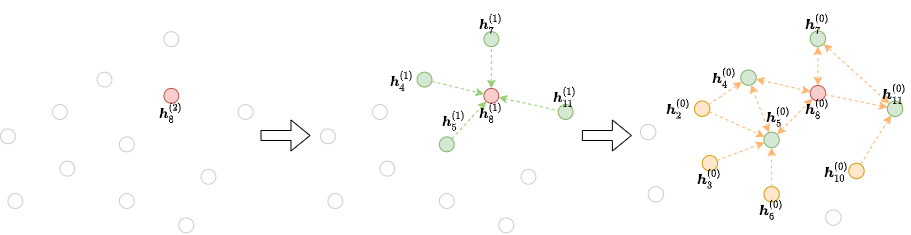# Chapter 6: Stochastic Training on Large Graphs¶

(中文版)

If we have a massive graph with, say, millions or even billions of nodes or edges, usually full-graph training as described in Chapter 5: Training Graph Neural Networks would not work. Consider an $$L$$-layer graph convolutional network with hidden state size $$H$$ running on an $$N$$-node graph. Storing the intermediate hidden states requires $$O(NLH)$$ memory, easily exceeding one GPU’s capacity with large $$N$$.

This section provides a way to perform stochastic minibatch training, where we do not have to fit the feature of all the nodes into GPU.

## Overview of Neighborhood Sampling Approaches¶

Neighborhood sampling methods generally work as the following. For each gradient descent step, we select a minibatch of nodes whose final representations at the $$L$$-th layer are to be computed. We then take all or some of their neighbors at the $$L-1$$ layer. This process continues until we reach the input. This iterative process builds the dependency graph starting from the output and working backwards to the input, as the figure below shows:With this, one can save the workload and computation resources for training a GNN on a large graph.

DGL provides a few neighborhood samplers and a pipeline for training a GNN with neighborhood sampling, as well as ways to customize your sampling strategies.